Courses

# Theory Of Machines And Vibrations - 2

## 20 Questions MCQ Test Mock Test Series - Mechanical Engineering (ME) for GATE 2020 | Theory Of Machines And Vibrations - 2

Description
This mock test of Theory Of Machines And Vibrations - 2 for GATE helps you for every GATE entrance exam. This contains 20 Multiple Choice Questions for GATE Theory Of Machines And Vibrations - 2 (mcq) to study with solutions a complete question bank. The solved questions answers in this Theory Of Machines And Vibrations - 2 quiz give you a good mix of easy questions and tough questions. GATE students definitely take this Theory Of Machines And Vibrations - 2 exercise for a better result in the exam. You can find other Theory Of Machines And Vibrations - 2 extra questions, long questions & short questions for GATE on EduRev as well by searching above.
QUESTION: 1

### The differential for a rear of driven vehicle is shown below. If the drive shaft turns 1000 rpm, then calculate the speed of the vehicle such that wheel does not slip. Take outside diameter of the wheels as 1 m.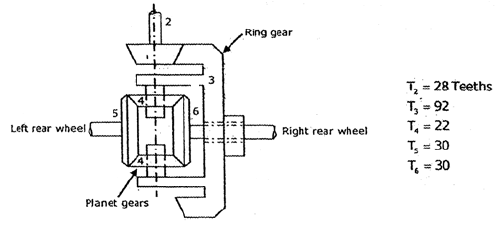Solution:

We know that,
ω3 /1 = ω6 /1

Given, ω= 1000 rpm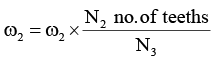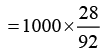= 304.374 rpm

Gear 3 is fixed to the carrier of the planetary drive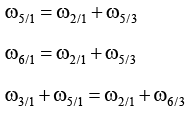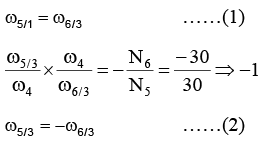Both 1 and 2 will be satisfies only when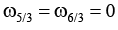Therefore,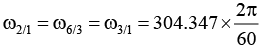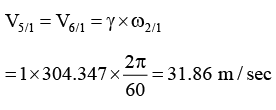QUESTION: 2

### In a four bar linkage mechanism, the input link has a radius of 25 cm and torque of 100 N.m, the output link has a length of 70 cm and a torque of 250 N.m. The mechanical advantage of the four bar linkage is

Solution:

Given rin = 25 cm rout = 750 cm

Tin = 100 Nm Tout = 250 Nm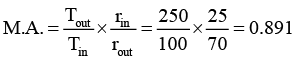QUESTION: 3

### To transmit energy of 10000 N.m with 5% coefficient of fluctuation, calculate mass moment of inertia of the system. The maximum and minimum fluctuation of energies is 750 Nm and 250 Nm respectively.

Solution:

E = 10,000
K = 5% = 0.05
ωmax = 750 ωmin = 250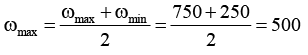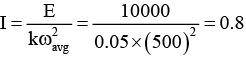QUESTION: 4

Match the following

Section – A
a. S + L < P + Q
b. S + L = P + Q
c. S + L > P + Q

​Section – B
1. Grashoff class – I type
2. Kutzbach class – I type
3. Grashoff class – II type
4. Kutzbach class – II type
5. Kutzbach class – III type
6. Grashoff class – III type

Solution:
QUESTION: 5

The mobility of the following linkage system is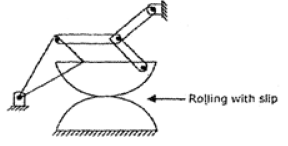Solution:

No. of links (N) = 6

No. of bars joint (j1) = 7

No. of higher joint (j1 ) = 1

DOF = 3 ( N − 1)− 2j1 − j2

= 3 6 − 1) − 2(7) − 1 = 0

QUESTION: 6

A mass of 20 kg is suspended form a spring which deflects 15 mm under this load. The body is subjected to a disturbing force with a maximum value of 100 N making 8 cycles/second. Find the amplitude of the ultimate motion.

Solution:

ω = 2π× f = 2π× 8 = 50.3 rad / s

Stiffness ( K ) =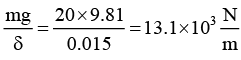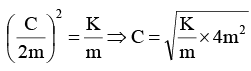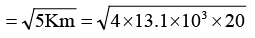= 1023 N / m / s

QUESTION: 7

Match the following
List – I
P. Ratio of force transmitted to force applied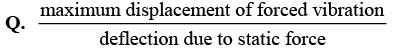R. Damping force per unit velocity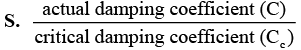List – II

1. Dimpling coefficient
2. Damping factor
3. Magnification factor
4. Logarithmic decrement
5. Transmissibility ratio

Solution:
QUESTION: 8

A simply supported shaft of 20 mm diameter and 0.6 m long carries a mass of 1 kg at its mid point. The density of the shaft is 40 × 103 kg/m3 and Young’s modulus of the shaft is 200 GPa. The critical speed of the shaft is __________ rpm.

Solution: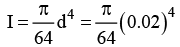= 7.855 ×10−9 m2 Mass of shaft per unit length (m1) = area × length × density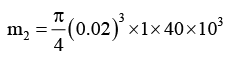= 12.6 kg / m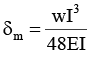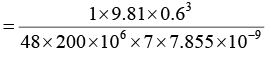= 0.133 × 10-3m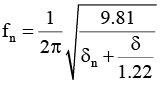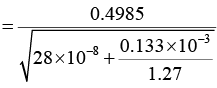= 43.3Hz

⇒ N = 43.3 × 60= 2598 rpm

QUESTION: 9

The measurements on a mechanical vibrating system show that it has a mass of 10 kg and that the springs can be combined to give an equivalent stiffness of the springs 6 N/mm. The dashpot is attached to the system which exerts a force of 40 N when the mass has a velocity of 1 m/s

Q. Determine the damping factor

Solution:

Given : C =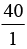= N / m / s;  Ct 2mωn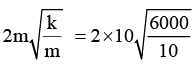= 498.898 N / m / s

Damping factor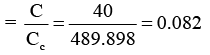QUESTION: 10

The measurements on a mechanical vibrating system show that it has a mass of 10 kg and that the springs can be combined to give an equivalent stiffness of the springs 6 N/mm. The dashpot is attached to the system which exerts a force of 40 N when the mass has a velocity of 1 m/s

Q. Determine the logarithmic decrement

Solution:

Logarithmic decrement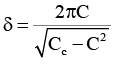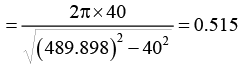QUESTION: 11

The degree of the freedom for the figure shown below is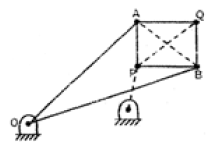Solution:

n = 3 ( N − 1) − 23
= 3 (8 − 1) − 2 ×10 = 21 − 20, n = 1

QUESTION: 12

A punching machine punches 3.8 cm diameter holes in a 3.2 cm thick plate and does 1000 J of work per square cm of sheared area. The punch has a stroke of 15 cm and punches 10 holes per minute. The energy required per punch is

Solution:

A3 = πdt = π× 3.8 × 3.2

A3 = 38.2 cm2

W.D = W.D / shear area × A5

= 1000 J / cm2 × 38.2 cm2= 38.2 kJ

QUESTION: 13

Which one of the following is correct?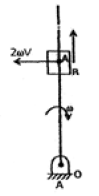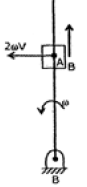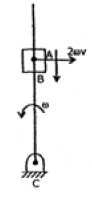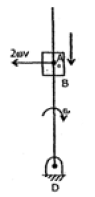Solution:
QUESTION: 14

An assemble of links and joints, interconnected in a way to provide a controlled output motion in response to a supplied input motion is defined as

Solution:
QUESTION: 15

A diameteral pitch of 6cm, 20° pressure angle, 19-tooth pinion is meshed with a 37-tooth gear. Determine the base pitch measured on the base circle.

Solution:
QUESTION: 16

Match the following

Section – A
a. Belt and rope drive with slip
b. Belt and rope drive without slip
c. Length of tooth parallel to gear axis
d. Watts indicator mechanism

Section – B

1. Face
2. Face width
3. Higher pair
4. Lower pair
5. Double crank mechanism
6. Double lever mechanism

Solution:
QUESTION: 17

If damping coefficient and critical damping coefficient of a vibrating system are 40N/m/s and 416 N/m/s respectively, then the logarithmic decrement is _______.

Solution: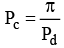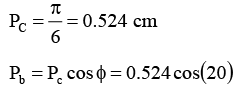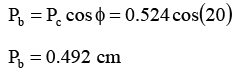QUESTION: 18

The two gear tooth 80 (pinion) and 30 (gear) are in contact with each other. The path of approach between the gears is 120 mm and path of recess is 80 mm. The angular velocity and diameter of pinion is 20 rad/s and 100 mm respectively.

Q. Determine the ratio of sliding velocity to rolling velocity at the beginning of contact.

Solution: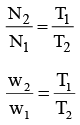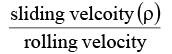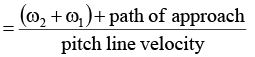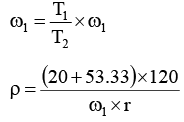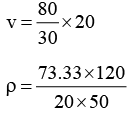ρ = 8.8

QUESTION: 19

The two gear tooth 80 (pinion) and 30 (gear) are in contact with each other. The path of approach between the gears is 120 mm and path of recess is 80 mm. The angular velocity and diameter of pinion is 20 rad/s and 100 mm respectively.

Q. Determine the ratio of sliding velocity to rolling velocity at the pitch point.

Solution: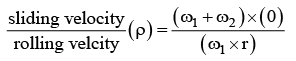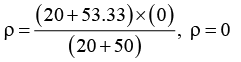QUESTION: 20

In an epicyclic gear train with a sun gear, planet gear and a moving arm. The ratio of number of teeth of the sun gear to that of the planet gear is 3. If the planet gear is fixed and the arm has an angular velocity of 150 RPM, the sun gear will rotate with ________ RPM.

Solution: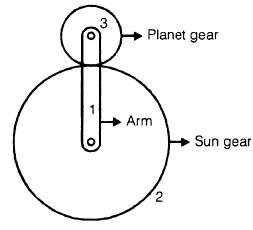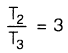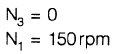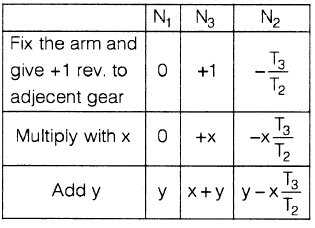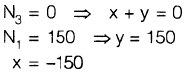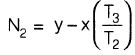|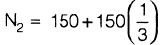= 150 + 50 = 200 rpm